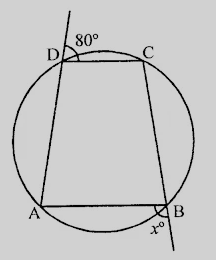"
">

# In the figure, $ABCD$ is a cyclic quadrilateral. Find the value of $x$."

Given:

$ABCD$ is a cyclic quadrilateral.

To do:

We have to find the value of $x$.

Solution:

$\angle CDE + \angle CDA = 180^o$            (Linear pair)

$80^o + \angle CDA = 180^o$

$\angle CDA = 180^o - 80^o$

$= 100^o$

In cyclic quadrilateral $ABCD$,

External $\angle B = Interior\ opposite\ angle\ CDA$

$= 100^o$

Therefore,

$x = 100^o$

The value of $x$ is $100^o$.

Updated on: 10-Oct-2022

46 Views